Perhaps you’ve heard that you should not reuse passwords but don’t understand why. After all, you have a really good password, one that nobody would ever guess, so why not use it everywhere?

First of all, people who think they have a really good password are usually wrong. They implicitly assume that an attacker would have to recreate the devious process they went through to create the password. This is not the case. A password needs to be hard for an attacker to crack, not hard for the owner to create, and these are not the same thing. The former requires passwords that are long and randomly generated.

## Credential stuffing

Now suppose you use a different username at the two sites. This is better, but the hack on X still means that your password has been added to a list of known passwords.

The worst case scenario is a site that stores passwords in the clear. This practice has long been discouraged, but the more times you reuse a password, the higher the chance that you’ll use your password at a site that does not hash passwords.

## Hashing

Most sites these days don’t store your password per se but a cryptographic hash of your password. When you enter your password, the site hashes it and compares the result to the hashed value it has on file. If they match, you’re in.

If your hashed password for X is part of a breach, and the hashing algorithms for X and Y known, an attacker can try hashing a list of known passwords and maybe one of them will match the hash of your password on Y.

If sites X and Y use different hashing algorithms, then a hash of your password for X is not directly useful to hacking your account on site Y. But it is possible to “unhash” passwords, especially if a site uses a hashing algorithm that has been broken. This takes a lot of computation, but people do it.

## Example

This is not hypothetical. It has happened many times. For example, it was part of what lead to the recent 23andMe breach. And when a hacker obtains one person’s family tree data, they obtain data on many other people at the same time. If you used a unique, long, randomly generated password on 23andMe but your cousin used password123, then your data may have been stolen.

## What to do?

What can you do? You almost have to use a password manager. A strong password is necessarily hard to remember, and memorizing hundreds of strong passwords would be quite a chore. Still, you might want to memorize one or two strong passwords if they protect something really valuable.

# The world’s sneakiest substitution

Something that seems like an isolated trick may turn out to be much more important. This is the case with a change of variables discovered by Karl Weierstrass.

Every calculus student learns a handful of standard techniques: u-substitutions, partial fractions, integration by parts, and trig substitutions. Then there is one more technique that is like something off a secret menu, something know only to the cognoscenti: Weierstrass’ t = tan(x/2) trick . Michael Spivak called this “the world’s sneakiest substitution.”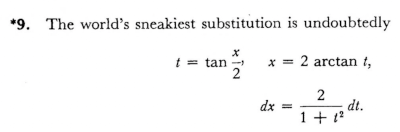This was on page 325 of the first edition of Spivak’s Calculus, page 360 of the second edition.

This innocent but apparently unmotivated change of variables has the following magical consequences:

• sin(x) = 2t/(1 + t2)
• cos(x) = (1 – t2) / (1 + t2)
• dx = 2 dt/(1 + t2).

This means that the trick can convert an integral containing any rational combination of trig functions into one involving only rational functions, which then can (in principle) be integrated in closed form using partial fractions. It’s the key that unlocks all trig integrals.

However, this is not as practical as it sounds. You may get a high-degree rational function, and while in theory the rational function can be integrated using partial fractions, the decomposition may be tedious if not impossible. Still, it’s interesting that a single trick reduces one large class of integration problems to another.

Now let’s leave integration behind. The equations above say that as t varies, the functions 2t/(1 + t2) and (1 – t2) / (1 + t2) take on all the values that sin(x) and cos(x) do. This means, for example, that you can draw a circle using graphics hardware that does not support sines and cosines. In theory the range of t would have to be infinite, but in practice the range would only have to be large enough to fill in the right pixels.

If we can parameterize a circle with rational functions, what else can we parameterize this way? It turns out, for example, that elliptic curves do not have rational parameterizations. This question is its own niche of algebraic geometry. I don’t know the history here, but it’s plausible some of this math was motivated by wondering what else could be done along the lines of Weierstrass’ trick.

 When I’ve mentioned this trick before, some have told me this was a routine part of their course. That was not my experience, either as a student or as an instructor. As a freshman I learned the trick from a friend who was the best of our cohort at integration, someone who would have done well at an integration bee if we’d had one. I felt I’d been let in on a secret.

# f(g(x)) versus g(f(x))

I stumbled upon a theorem today that I feel like I’ve needed in the past, though I can’t remember any particular applications. I’m writing it up here as a note to my future self should the need reappear.

The theorem gives sufficient conditions to conclude

f(g(x)) ≤ g(f(x))

and uses this to prove, for example, that

arcsin( sinh(x/2) ) ≤ sinh( arcsin(x)/2 )

on the interval [0, 1].

Here’s the theorem, found in .

Let f be continuous with domain 0 ≤ x < 1 or 0 ≤ x ≤ 1, f(0) = 0, f(1) > 1 (including the possibility that f(1) = +∞); let g be continuous with domain the range of f, and g(1) ≤ 1. Let f(x)/x and g(x)/x be strictly increasing on their domains. Finally let f(x) ≠ x for 0 < x < 1. Then f(g(x)) ≤ g(f(x)) for 0 < x < 1.

 Ralph P. Boas. Inequalities for a Collection. Mathematics Magazine, January 1979, Vol. 52, No. 1, pp. 28–31

# Constellations in Mathematica

Mathematica has data on stars and constellations. Here is Mathematica code to create a list of constellations, sorted by the declination (essentially latitude on the celestial sphere) of the brightest star in the constellation.

```constellations = EntityList["Constellation"]
sorted = SortBy[constellations, -#["BrightStars"][]["Declination"] &]
```

We can print the name of each constellation with

`Map[#["Name"] &, sorted]`

This yields

```{"Ursa Minor", "Cepheus", "Cassiopeia", "Camelopardalis",
…, "Hydrus", "Octans", "Apus"}
```

We can print the name of the constellation along with its brightest star as follows.

```Scan[Print[#["Name"], ", " #["BrightStars"][]["Name"]] &, sorted]
```

This prints

```Ursa Minor, Polaris
Cepheus, Alderamin
Cassiopeia, Tsih
Camelopardalis, β Camelopardalis
…
Hydrus, β Hydri
Octans, ν Octantis
Apus, α Apodis
```

Mathematica can draw star charts for constellations, but when I tried

`Entity["Constellation", "Orion"]["ConstellationGraphic"]`

it produced extraneous text on top of the graphic.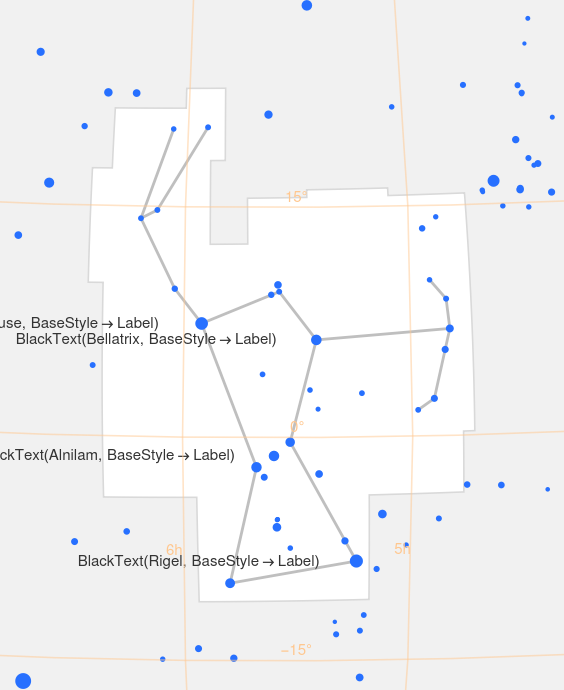# 222nd Carnival of Mathematics

A blog carnival is a round up of recent blog posts. The Carnival of Mathematics is a long-running carnival of blog posts on mathematical topics. This is the 222nd edition of the carnival.

By longstanding tradition, the nth Carnival of Mathematics must begin with trivia about the number n, and so here are five facts about the number 222.

• There are six groups of order 222, and 222 groups of order 912.
• 222=9+87+6×5×4+3+2+1.
• You can encode the number 222 in the Major mnemonic system as “unknown” or “one-on-one.”

## The posts

Gil Kalai looks at what happens when you ask ChatGPT to solve Elchanan Mossel’s dice problem.

Γιώργος Πλούσος (@plousos2505 on the social media platform formerly know as Twitter) posted an image showing how to compute π via a sequence of approximations requiring only basic geometry.

David Eppstein’s blog 11011110 has a post on Pyramidology. It’s particularly fitting to have a post from 1101110 in this carnival. As the author explains in his About page,

This journal’s name comes from interpreting my initials as the hexadecimal number 0xDE (decimal 222) and then converting the result to binary.

The blog ThatsMaths has a post on Sharkovsky numbering, Oleksandr Sharkovsky (1936–2022), and Sharkovsky’s Theorem. This post includes the beautiful image from Wikimedia.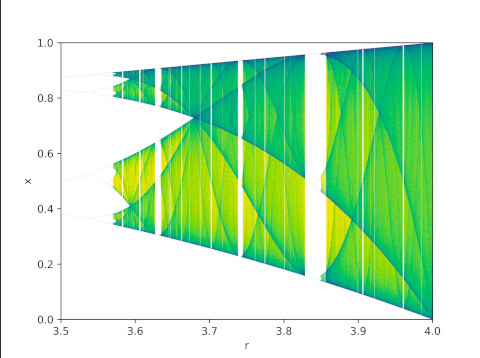The post Patterns of reality by Timothy Williamson gives an overview of logic from basics to the work of Gödel and Turing.

Larissa Fedunik-Hofman posts an interview with Andrew Krause discussing dynamical system.

Finally, we have Matthew Scroggs’ Advent calendar of math puzzles.

To see previous editions of the carnival, or to submit a post to the next edition, see the Carnival of Mathematics page on Aperiodical.

# Bounding complex roots by a positive root

Suppose you have an nth degree polynomial with complex coefficients

p(z) = anzn + an-1zn-1 + … + a0

and you want to find some circle that is guaranteed to contain all the zeros of p.

Cauchy found such a circle in 1829. The zeros of p lie inside the circle |z| ≤ r where r is the unique positive root of

f(z) = |an|zn − |an-1|zn-1 − … − |a0|

This value of r is known as the Cauchy radius of the polynomial p.

This may not seem like much of an improvement: you started with wanting to find the roots of an nth degree polynomial and you end with finding the roots of an nth degree polynomial. But Cauchy’s theorem reduces the problem of finding all roots of a complex polynomial to finding one root of a real polynomial. Furthermore, the positive root we’re after is guaranteed to be unique.

If a0 = 0 then p(z) has a factor of z and so we can reduce the problem to bounding the zeros of p(z)/z. Otherwise, f(0) < 0. Eventually f(z) must be positive because the zn term will overtake the rest of the terms for large enough z. So we only need to find some value of z where f(z) > 0 and then we could use the bisection method to find r.

Since our goal is to bound the zeros of p, we don’t need to find r exactly: an upper bound on r will do, though the smaller the upper bound the better. The bisection method gives us a sequence of upper bounds, so we could work in rational arithmetic and have rigorously provable upper bounds.

As for how to find a real value of z where f is positive, we could try z = 2k for successive value of k until we find one that works.

For example, let’s bound the roots of

p(z) = 12z5 + 2z2 + 23i = 0.

Cauchy’s theorem says we need to find the unique positive root of

f(z) = 12z5 − 2z2 − 23.

Now f(0) = −23 and f(2) = 353. So we know right away that the roots of p have absolute value less than 2.

Next we evaluate f(1), which turns out to be −13, and so the Cauchy radius is larger than 1. This doesn’t necessarily mean that p has a root with absolute value greater than 1, only that the Cauchy radius is greater than 1. An upper bound on the Cauchy radius is an upper bound on the absolute values of the roots of p; a lower bound on the Cauchy radius is not necessarily a lower bound on the largest root.

Carrying out two steps of the bisection method by hand was easy, but let’s automate the process of carrying it out further.

```>>> from scipy.optimize import bisect
>>> bisect(lambda x: 12*x**5 - 2*x*x - 23, 1, 2)
1.1646451258329762```

So Python tells us r = 1.1646451258329762.

Here’s a plot of the roots and the Cauchy radius.In this example the roots of p are located very near a circle with the Cauchy radius. The roots range in absolute value between 1.1145600699993699 and 1.1634197192917954. The roots nearly lie in a circle because the quadratic term in our polynomial is small and so we are approximately finding the fifth roots of −23i.

Let’s do another example with randomly generated coefficients to get a better idea of how Cauchy’s theorem works in general. The coefficients of our polynomial, from 0th to 5th, are

0.126892 + 0.689356i,  -0.142366 + 0.260969, – 0.918873 + 0.489906i,  0.0599824 – 0.679312i,  – 0.222055 + 0.273651, + 0.154408 + 0.733325i

The roots have absolute value between 0.7844606228243709 and 1.2336256274024142, and the Cauchy radius is 1.5088421845957782. Here’s a plot.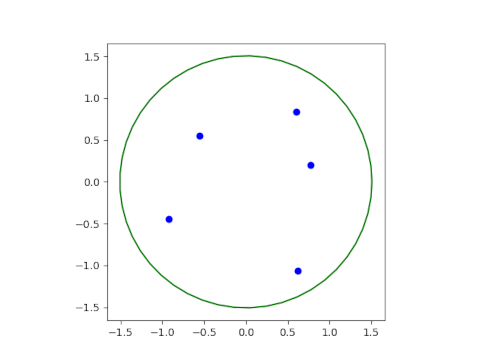# Convergent subsequence

I was reading a theorem giving conditions for a divergent series to have a convergent subseries and had a sort of flashback.

I studied nonlinear PDEs in grad school, which amounted to applied functional analysis. We were constantly proving or using theorems about sequences having convergent subsequences, often subsequences that converged in a very weak sense.

This seemed strange to me at first. If a sequence diverges, why is it of any interest that a subsequence converges? This seemed like blackout poetry, completely changing the meaning of a text by selecting various words. For example, here is the opening paragraph of Pride and Prejudice, blacked out to appear to be a real estate ad.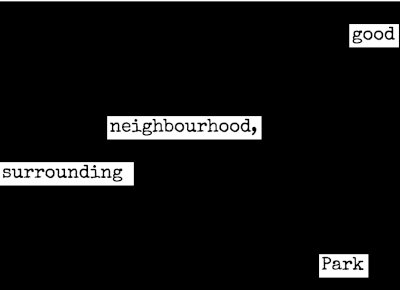Here’s the big picture I was missing. We’re trying to show that a differential equation has a solution, and we’re doing that by some kind of successive approximation. Maybe our series of approximations doesn’t work in general, but that doesn’t matter. We’re just trying to find something that is a solution. Once you come up with a candidate solution, by whatever means, grasping at whatever straws you can grasp, you then prove that the candidate really is a solution, perhaps a solution in a weak sense. Then you show that this solution, potentially one of many, is unique. Then you show that your weak solution is a in fact a solution in a stronger sense.

# How to memorize the periodic table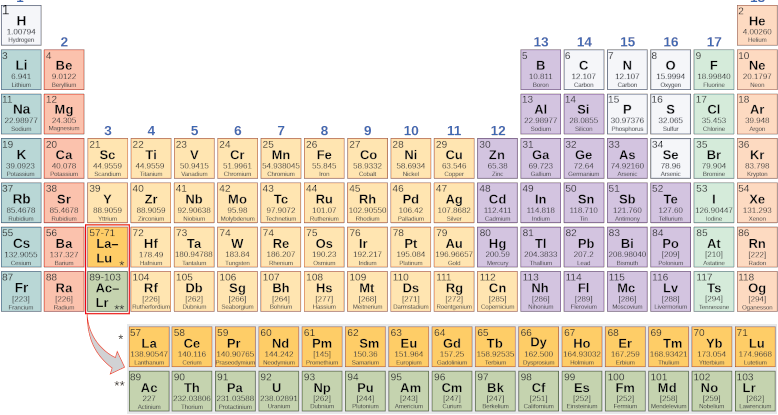## Motivation

Memorizing the periodic table has some practical value, especially if you’re a chemist, but in any case it’s an interesting exercise, easier to do than it may sound. And it’s a case study for how you might memorize other things of more practical value to you personally.

## Major system pegs

The Major system is a way to associate consonant sounds to numbers. You can fill in vowels and semivowels as you please to turn the sequence of consonant sounds into words, preferably words that create a vivid image in your mind.

You can pick a canonical encoding of each number to create a set of pegs and use these to memorize numbered lists. Although numbers can be encoded many ways, a set of pegs is a one-to-one mapping to numbers. To pull up the nth item in the list, recall what image you’ve associated with the peg image for n.

For example, you could encode 16 as dish, tissue, touché, Hitachi, etc. If you want to remember that sulfur has atomic number 16 you could use any of those images. But if you wanted to remember that the 16th element is sulfur, you need to have a unique peg associated with 16.

Learning pegs is more work than hanging things on pegs. But once you have a set of pegs, you can reuse them for memorizing multiple lists. For example, you could use the same pegs to memorize the periodic table and the ASCII table.

## Atomic numbers

Allan Krill has written up a way to associate each element with a peg. You could use his suggestions, but you’ll almost certainly need to customize some of them. It’s generally hard to use anyone else’s mnemonics. What works for one person may not for another.

To memorize the periodic table, you first come up with pegs for the numbers 1 through 118. Practice those and get comfortable with them. This could take a while, but it’s reusable effort. Then associate an image of each element with its corresponding peg. For example, polonium is element 84. If your peg for 84 is fire, you might imagine someone playing polo on a field that’s on fire.

## Element symbols

Every element has a one- or two-letter symbol, and most of these are easy: Ti for titanium, U for uranium, etc. Some seem completely arbitrary, such as Hg for mercury, but these you may already know. These names seem strange because they are mnemonic in Latin. But the elements with Latin names are also the ones that were discovered first and are the most common. You probably know by osmosis, for example, that the symbol for iron is Fe.

The hard part is the second letter, if there is a second letter. For example, is does Ar stand for argon or arsenic? Is the symbol for thulium Th or Tl or Tm?

When you associate an element image with a peg image, you could add a third image for the second letter of the element symbol, using the NATO phonetic alphabet if you know that. For example, the NATO word for S is Sierra. If your peg for 33 is mummy, you might imagine a mummy drinking a bottle of Sierra Springs® water laced with arsenic.

# Solving a triangle the size of Argentina

The numbers in today’s date—11, 28, and 23—make up the sides of a triangle. This doesn’t always happen; the two smaller numbers have to add up to more than the larger number.

We’ll look at triangles with sides 11, 23, and 28 in the plane, on a sphere, and on a hypersphere. Most of the post will be devoted to the middle case, a large triangle on the surface of the earth.

## Solving a triangle in the plane

If we draw a triangle with sides 11, 23, and 28, we can find out the angles of the triangle using the law of cosines:

c² = a² + b² – 2ab cos C

where C is the angle opposite the side c. We can find each of the angles of the triangle by rotating which side we call c.

If we let c = 11, then C = arccos((23² + 28² − 11²)/(2 × 23 × 28)) = 22.26°.

If we let c = 23, then C = arccos((11² + 28² − 23²)/(2 × 11 × 28)) = 52.38°.

If we let c = 28, then C = arccos((11² + 23² − 28²)/(2 × 11 × 23)) = 105.36°.

## Solving a triangle on a sphere

Now suppose we make our 11-23-28 triangle very large, drawing our triangle on the face of the earth. We pick our unit of measurement to be 100 miles, and we get a triangle very roughly the size and shape of Argentina.

We can still use the law of cosines, but it takes a different form, and the meaning of the terms changes. The law of cosines on a sphere is

cos(c) = cos(a) cos(b) + sin(a) sin(b) cos(C).

As before, a, b, and c are sides of the triangle, and the sides b and c intersect at an angle C. However, now the sides themselves are angles because they are arcs on a sphere. Now a, b, and c are measured in degrees or radians, not in miles.

If the length of an arc is x, the angular measure of the arc is 2πx/R where R is the radius of the sphere. The mean radius of the earth is 3959 miles, and we’ll assume the earth is a sphere with that radius.

We can solve for the angle opposite the longest side by using

C = arccos( (cos(c) – cos(a) cos(b)) / sin(a) sin(b) )

where

a = 2π × 1100 / 3959
b = 2π × 2300 / 3959
c = 2π × 2800 / 3959

It turns out that C = 149.8160°, and the other angles are 14.3977° and 29.4896°.

Importantly, the sum of these three angles is more than 180°. In fact it’s 193.7033°.

The sum of the vertex angles in a spherical triangle is always more than 180°, and the bigger the triangle, the more the sum exceeds 180°. The amount by which the sum exceeds 180° is called the spherical excess E and it is proportional to the area. In radians,

E = area / R².

In our example the excess is 13.7033° and so the area of our triangle is

13.7033° × (π radians / 180°) × 3959² miles² = 3,749,000 miles².

Now Argentina has an area of about a million square miles, so our triangle is bigger than Argentina, but smaller than South America (6.8 million square miles). Argentina is about 2300 miles from north to south, so one of the sides of our triangle matches Argentina well.

Note that there are no similar triangles on a sphere: if you change the lengths of the sides proportionately, you change the vertex angles.

## Solving a triangle on a pseudosphere

In a hyperbolic space, such as the surface of a pseudosphere, a surface that looks sorta like the bell of a trombone, the law of cosines becomes

cosh(c) = cosh(a) cosh(b) + κ sinh(a) sinh(b) cos(C)

where κ < 0 is the curvature of the space. Note that if we set κ = 1 and delete all the hs this would become the law of cosines on a sphere.

Just as the sum of the angles in a triangle add up to more than 180° on a sphere, and exactly 180° in a plane, they add up to less than 180° on a pseudosphere. I suppose you could call the difference between 180° and the sum of the vertex angles the spherical deficiency by analogy with spherical excess, but I don’t recall hearing that term used.

# Unix linguistics

If you knew that you wanted to learn 10 spoken languages, it would probably be helpful to take a course in linguistics first. Or maybe to have a linguistics course after learning your first or second language. And if the languages are related, it would help to know something about the linguistics of that group of languages in particular. For example, if you wanted to learn several Romance languages, it might be worthwhile to learn at least some Latin first, even if Latin isn’t on the list of languages you want to learn.

In order to become fluent in using the Unix (Linux) command line, you need to learn a dozen related languages. Fortunately none of these languages are anywhere near as large as a spoken language, but there are several of them. Regular expressions, for example, are a pattern description language. You can think of vim as a language. And of course programming languages like `sed` and `awk` are languages.

As you use various command line utilities you notice similarities between them. Some tool history is fairly well known. For example, it’s well known that `grep` takes its name from the `g/re/p` command in `ed`, and that `grep` was created by modifying the `ed` source code. The history of `sed` is similar. The line editor `ed` is a common ancestor of `grep`, `sed`, and `vi`, which explains a lot of the similarity between these tools.

There is a large amount of preserved Unix history, but what I have in mind is more linguistics than history. History often accounts for the similarities in syntax, but I’m primarily interested in the similarities themselves rather than the history. A semi-fictional history might be more useful than an accurate history. “This isn’t exactly how things came about, but you could imagine …”

I’ve seen bits and pieces of what I imagine would comprise a course in Unix linguistics. For example, there is a section in the book sed & awk entitled “Awk, by Sed and Grep, out of Ed.”

I’ve used Emacs since college, but I’m learning how to get by in `vi`. Part of my motivation is to be able to log into a client’s Linux box and be productive without being able to install or configure anything. Although I hardly know anything about `vi` at this point, I can tell right away that `vi` has more syntactic similarity to the rest of the Unix ecosystem than Emacs does.

It would be really nice to have a book with a title like “`vi` for people who have used `sed`, `grep`, and `less`.” Or even better, a tutor who could relate what I don’t know to what I do know. Someone like my Greek professor.

I took one semester of classical Greek in college. The professor, William Nethercut, was amazing. At the beginning of the semester he asked each student what languages they had studied, and customized the rest of the course accordingly. “This feature of Greek is like that feature in French, Susan. And like this feature of Latin, Mike.” I was impressed by his erudition in languages, but even more impressed with his thoughtfulness in relating to each of us individually. If Dr. Nethercut taught a class in the Unix ecosystem, he could say “So, you know this set of tools and want to learn that set of tools. You’ll find that the syntax of this is similar to the syntax of that, but watch out for this difference.”